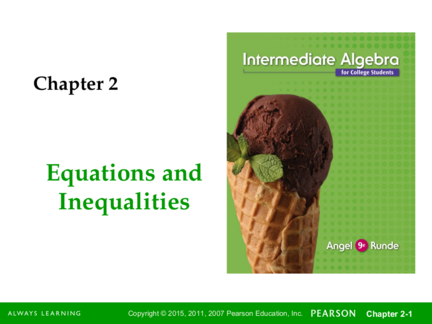# Equations and InequalitiesContributed by:The discussed topic in the ppt is: Solving Linear Equations
1. Chapter 2
Equations and
Inequalities
1
2. Chapter Sections
2.1 – Solving Linear Equations
2.2 – Problem Solving and Using Formulas
2.3 – Applications of Algebra
2.5 – Solving Linear Inequalities
2.6 – Solving Equations and Inequalities
Containing Absolute Values
2
3. § 2.1
Solving Linear
Equations
3
4. Properties of Equality
Properties of Equality
For all real numbers a, b, ,and c:
1. a = a Reflexive property
2. If a = b, then b = a Symmetric property
3. If a = b, and b = c, then a = c Transitive property
4
5. Combine Like Terms
Like terms are terms that have the same
variables with the same exponents.
Like Terms Unlike Terms
1
-3x, 8x, - 3 x 20x, x2, x3
6w2, -12w2, w2 6xy, 2xyz, w2
5
6. Combining Like Terms
1. Determine which terms are like terms.
2. Add or subtract the coefficients of the like
terms.
3. Multiply the number found in step 2 by the
common variable(s).
Example: 5a + 7a = 12a
6
7. Distributive Property
For any real numbers a, b, and c,
a(b + c) = ab + bc
Example: 3(x + 5) = 3x + 15
(This is not equal to 18x! These are
not like terms.)
7
8. Simplifying an Expression
1. Use the distributive property to remove
any parentheses.
2. Combine like terms.
Simplify 3(x + y) + 2y
= 3x + 3y + 2y (Distributive Property)
= 3x + 5y (Combine Like Terms)
(Remember that 3x + 5y cannot be combined because
they are not like terms.)
8
9. Solve Linear Equations
A linear equation in one variable is an
equation that can be written in the
form ax + b = c where a, b, and c are real
numbers and a  0.
The solution to an equation is the
number that when substituted for the
variable makes the equation a true
9
If a = b, then a + c = b + c for any
real numbers a, b, and c.
Example:
Solve the equation x – 4 = -10.
x – 4 = -10
x – 4 + 4 = -10 + 4 (Add 4 to both sides.)
x = -6
Check: (-6) – 4 = -10
10
11. Multiplication Property of Equality
If a = b, then a · c = b · c for any real
numbers a, b, and c.
Example: Solve the equation 12y = 15.
1 1 1
12 ·12y
1
= 15 · 12 (Multiply both sides by 12 )
5
1 12 y 15 1
12 12
1
y 5 4
4
11
12. Multiplication Property of Equality
Example: Solve the equation 1 x 3 .
4 4
( 4)( 1 x) 3 ( 4) (Multiply both sides by -4)
4 4
( 4)( 1 x) 3 ( 4) (Simplify)
4 4
x = -3
1 (-3) 3
Check: 4

4
12
13. Solve Linear Equations
1. Clear fractions. If the equation contains fractions, eliminate the fractions by
multiplying both sides of the equation by the least common denominator.
2. Simplify each side separately. Simplify each side of the equation as much as
possible. Use the distributive property to clear parentheses and combine like
terms as needed.
3. Isolate the variable term on one side. Use the addition property to get all
terms with the variable on one side of the equation and all constant terms on
the other side. It may be necessary to use the addition property a number of
times to accomplish this.
13
14. Solve Linear Equations
4. Solve for the variable. Use the multiplication
property to get the variable (with a coefficient
of 1) on one side.
5. Check. Check by substituting the value
obtained in step 4 back into the original
equation.
14
15. Solving Equations
Example: Solve the equation 2x + 9 = 14.
2 x  9 14
2 x  9  9 14  9
2 x 5
2x 5

2 2
5
x
2 Don’t forget to check!
15
16. Solve Equations Containing Fractions
2a
Example: Solve the equation 5   9
3
The least common denominator is 3.
2a
5  9 15  15  2a  27  15
3
 2a  42
 2a 
3 5   3( 9)  2a  42
 3 
2 2
 2a 
3(5)  3   27 a 21
 3
15  2a  27
16
and Identities
Conditional Equations: Equations that true for only specific
values of the variable.
Contradictions: Equations that are never true and have no solution.
Identities: Equations that are always true and have an infinite
number of solutions.